### Home > CAAC > Chapter 7 > Lesson 7.3.4 > Problem7-116

7-116.

Copy and complete each of the Diamond Problems below. The pattern used in the Diamond Problems is shown at right.1.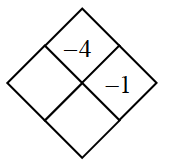•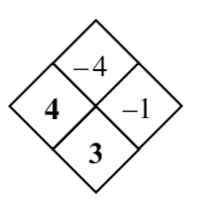1.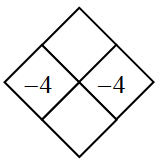•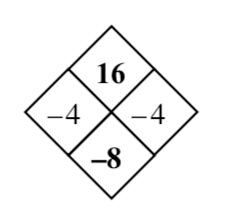1.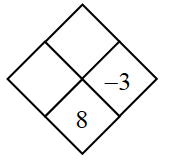• What plus $−3$ equals $8$?
What does $−3$ multiplied by that number equal?

1.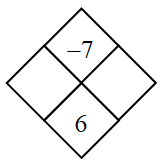• What are the factors of $−7$? Which two added together equal $6$?